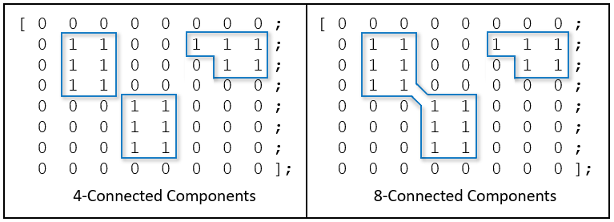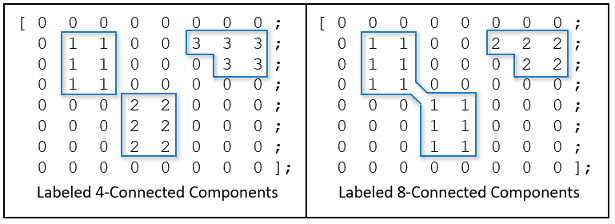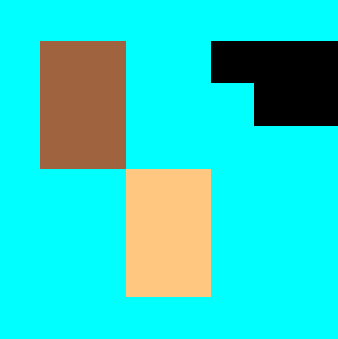## Label and Measure Connected Components in a Binary Image

### Detect Connected Components

A connected component, or an object, in a binary image is a set of adjacent pixels. Determining which pixels are adjacent depends on how pixel connectivity is defined. For a two-dimensional image, there are two standard connectivities:

• 4-connectivity — Pixels are connected if their edges touch. Two adjoining pixels are part of the same object if they are both on and are connected along the horizontal or vertical direction.

• 8-connectivity — Pixels are connected if their edges or corners touch. Two adjoining pixels are part of the same object if they are both on and are connected along the horizontal, vertical, or diagonal direction.

You can also define nonstandard connectivity, or connectivities for higher dimensional images. For more information, see Pixel Connectivity.

This figure shows two identical matrices that represent a binary image. On each matrix is an overlay highlighting the connected components using 4-connectivity and 8-connectivity, respectively. There are three connected components using 4-connectivity, but only two connected components using 8-connectivity.You can calculate connected components by using the `bwconncomp` function. In this sample code, `BW` is the binary matrix shown in the above figure. For this example, specify a connectivity of 4 so that two adjoining pixels are part of the same object if they are both on and are connected along the horizontal or vertical direction. The `PixelIdxList` field identifies the list of pixels belonging to each connected component.

```BW = zeros(8,8); BW(2:4,2:3) = 1; BW(5:7,4:5) = 1; BW(2,6:8) = 1; BW(3,7:8) = 1; BW```
```BW = 0 0 0 0 0 0 0 0 0 1 1 0 0 1 1 1 0 1 1 0 0 0 1 1 0 1 1 0 0 0 0 0 0 0 0 1 1 0 0 0 0 0 0 1 1 0 0 0 0 0 0 1 1 0 0 0 0 0 0 0 0 0 0 0```
```cc4 = bwconncomp(BW,4) ```
```cc4 = Connectivity: 4 ImageSize: [8 8] NumObjects: 3 PixelIdxList: {[6x1 double] [6x1 double] [5x1 double]} ```

For comparison, calculate the connected components of the same binary image using the default connectivity, 8.

```cc8 = bwconncomp(BW) ```
```cc8 = Connectivity: 8 ImageSize: [8 8] NumObjects: 2 PixelIdxList: {[12x1 double] [5x1 double]} ```

### Label Connected Components

Labeling connected component is the process of identifying the connected components in an image and assigning each one a unique label. The resulting matrix is called a label matrix.

This figure shows the two label matrices that label the connected components using 4-connectivity and 8-connectivity, respectively.Create a label matrix by using the `labelmatrix` function. This sample code continues with the connected component structure, `cc4`, defined in the preceding section.

`L4 = labelmatrix(cc4)`
```L4 = 8×8 uint8 matrix 0 0 0 0 0 0 0 0 0 1 1 0 0 3 3 3 0 1 1 0 0 0 3 3 0 1 1 0 0 0 0 0 0 0 0 2 2 0 0 0 0 0 0 2 2 0 0 0 0 0 0 2 2 0 0 0 0 0 0 0 0 0 0 0```

To visualize connected components, display the label matrix as a pseudo-color image by using the `label2rgb` function. The label identifying each object in the label matrix maps to a different color in the associated colormap. You can specify the colormap, background color, and how objects in the label matrix map to colors in the colormap.

```RGB_label = label2rgb(L4,@copper,"c","shuffle"); imshow(RGB_label)```### Select Objects in a Binary Image

You can use the `bwselect` function to select individual objects in a binary image. Specify pixels in the input image programmatically or interactively with a mouse. `bwselect` returns a binary image that includes only those objects from the input image that contain one of the specified pixels.

For example, use this command to select objects in the image displayed in the current axes.

`BW = bwselect;`

The cursor changes to cross-hairs when it is over the image. Click the objects you want to select; `bwselect` displays a small star over each pixel you click. When you are done, press Return. `bwselect` returns a binary image consisting of the objects you selected, and removes the stars.

### Measure Properties of Connected Components

The `regionprops` function can return measurements for several properties of connected components. Other functions measure a single property. For example, the `bwarea` function returns the area of a binary image.

This example uses `bwarea` to determine the percentage area increase in `circbw.tif` that results from a dilation operation. The area is a measure of the size of the foreground of the image and is roughly equal to the number of `on` pixels in the image. However, `bwarea` does not simply count the number of pixels set to `on`. Rather, `bwarea` weights different pixel patterns unequally when computing the area. This weighting compensates for the distortion that is inherent in representing a continuous image with discrete pixels. For example, a diagonal line of 50 pixels is longer than a horizontal line of 50 pixels. As a result of the weighting `bwarea` uses, the horizontal line has area of 50, but the diagonal line has area of 62.5.

```BW = imread('circbw.tif'); SE = ones(5); BW2 = imdilate(BW,SE); increase = (bwarea(BW2) - bwarea(BW))/bwarea(BW)```
```increase = 0.3456```